Surface Integrals over n-Dimensional Spheres by Stanislav Sýkora, Extra Byte, Via R.Sanzio 22C, Castano Primo, Italy 20022 in Stan's Library, Ed.S.Sykora, Vol.I. First release May 30, 2005 Permalink via DOI:  10.3247/SL1Math05.002
 Other math articles | Stan's Library Stan's LINKS and  Courses | Stan's HUB
 Abstract This is the second Note in a series  dealing with volume and surface integrals over n-dimensional ellipsoids. Here we evaluate integrals of products of powers of the Cartesian vector components over surfaces of n-dimensional, real spheres which can be expressed by explicit formulae (this, of course, includes the surface areas themselves). Previous Note in this series: Volume Integrals over n-Dimensional Ellipsoids. Next Note in this series: On the Circumference of Ellipses.

1. Introduction

In this Note we focus our attention on a class of surface integrals over n-dimensional real spheres which can be evaluated explicitly. We postpone the evaluation of surface integrals over generic ellipsoids because they involve transcendental elliptic integrals.

Like in , let Rn denote the n-dimensional Euclidean space, r the position vector in Rn and r = |r| its norm

(1)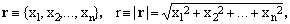where the x's are its Cartesian coordinates. We shall often use n-tuples of non-negative real exponents

(2)which, however, are not to be intended as elements of Rn.
The shorthand will be exploited in conventional expressions of the type

(3)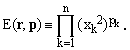In this notation, an n-dimensional spherical surface S(R) of radius R is defined by the condition

(4)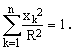We are interested in the evaluation of the following integrals over S(R):

(5)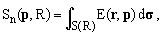where dσ is an (n-1)-dimensional surface element.

2. Evaluation of the integrals

Evaluation of the integrals (5) is considerably simplified by three facts:

a) From reference , Equation 17, we know the value of the corresponding volume integral over the n-dimensional sphere, which turns out to be

(6)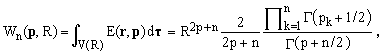where Γ(x) denotes the gamma function [4,5].

b) Integrals (5) have the nearly self-evident scaling property

(7)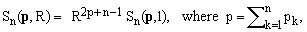arising from the fact that E(r,p) scales with 2p-th power of R and dσ scales with (n-1)-st power or R.

c) For spheres (unlike generic ellipsoids), the volume integration can be carried out by summing the contributions of concentric shells defined by radii r and r+dr, for r ranging from 0 to R. Hence

(8)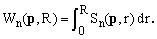From (8) and (7) it follows that

(9)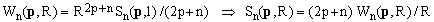from which, comparing with (6), we obtain the result

(10)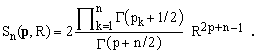In the original paper  which stimulated this series of Notes, formula (10) was derived in a more direct - and also more laborious - way. It is therefore pleasing to note that the two procedures lead to the same result.

3. Admissible values of the exponents (p's)

For reasons discussed in , the values of pk may assume any real value greater than -1/2, which are also the values compatible with Equation (10). Moreover, one should keep in mind that in equations (3) and (5), the individual Cartesian coordinates xk get first squared and only afterwards elevated to pk. Thus, for example, when pk = -1/4, the corresponding factor in Eq.(3) is the square root of |xk|.

4. Special cases

Setting all the p's equal to ν/2, one obtains the following formula, valid for any ν>-1:

(11)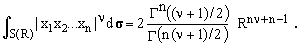In particular, setting ν = 1 and taking into account that Γ(n) = (n-1)!, we obtain the formula

(12)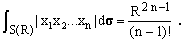When only one of the p's equals ν/2 and all the others are zero, Eq.(10) yields the identity, again valid for any ν>-1:

(13)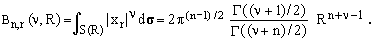For the important cases of ν=1 and ν=2, this gives

(14)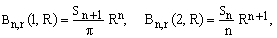where

(15)5. Surfaces of n-dimensional spheres

When all the p's are zero, Eq.(10) gives simply the surface of the n-dimensional sphere. Explicitly, this turns out to be

(16)showing that the the numbers Sn of Eq.(22) are the surfaces of n-dimensional spheres of unit radius whose values can be easily calculated by means of the recurrence

(17)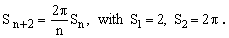The surface of an n-dimensional sphere has the dimension of the (n-1)-st power of length. This coincides with the popular notion of "surface area" only in the case of n=3 but its extension to any n is quite obvious. In particular, for n=2 it coincides with the common concept of "circumference" of a circle. The value of 2 for n=1, implicit in the above recurrence relations, can be considered as the cardinality of the set of the two end points of a 1D interval.

Surfaces and other surface integrals for n-dimensional spheres with unit radius

 Dim Surface Surface integral of: symbolic numeric |x1| x12 |x1x2| x12x22 1 2 2.000000.. 2 2 - - 2 2π 6.283185.. 4 π 2 π/4 3 4π 12.566370.. 2π 4π/3 8/3 4π/15 4 2π2 19.739208.. 8π/3 π2/2 π π2/12 5 8π2/3 26.318945.. π2 8π2/15 16π/15 8π2/105 6 π3 31.006276.. 16π2/15 π3/6 π2/3 π3/48 7 16π3/15 33.073361.. π3/3 16π3/105 32π2/105 16π3/1185 8 π4/3 32.469697.. 32π3/105 π4/24 π3/12 π4/240 9 32π4/105 29.686580.. π4/12 32π4/945 64π3/945 32π4/10395 10 π5/12 25.501640.. 64π4/945 π5/120 π4/60 π5/1440

Note: Numerically, the surface integral of x12  over a unit sphere equals its volume.

6. Asymptotic behavior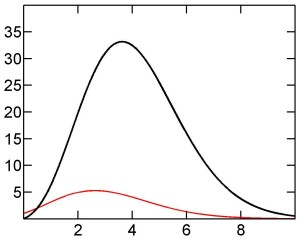Equation (15) for the surface of an n-dimensional sphere of unit radius can be rewritten in terms of the following function σ(x):

(18)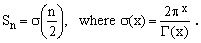whose graph is shown on the right (bold black line), together with the analogous function ν(x) for unit sphere volumes (thin red line). The relation between the two functions is σ(x) = 2x.ν(x).

It is evident that both functions pass through a maximum and then, for large x, decay rapidly to zero. A very good approximation for the logarithms of both functions can be obtained by a straightforward application of Stirling formula [4,5] for ln(Γ(x)).

The asymptotic behavior is rather complicated. Roughly, one can affirm that, for very large x, the decay of both functions is dominated by a factor of the form O[x-x].

 References Sykora S., Volume Integrals over n-Dimensional Ellipsoids, in Stan's Library, Ed.S.Sykora, Vol I, 2005. Sykora S., Surface Integrals over n-Dimensional Spheres, in Stan's Library, Ed.S.Sykora, Vol I, 2005. (URL of this Note). Sykora S., Quantum Theory and the Bayesian Inference Problems, J.Statistical Phys. 11, 17 (1974). Abstract. Abramowitz M., Stegun A., Editors, Handbook of Mathematical Functions, National Bureau of Standards 1964, Dover Publications, New York 1972. John Wiley & Sons, Reprint edition 1993. Arfken G.B., Weber H., Mathematical Methods for Physicists, Academic Press, 5th edition 2000.
 TOP | Other math articles | Stan's Library Stan's LINKS and  Courses | Stan's HUB | TOP